Nonlinear Differential Equations,
Electron. J. Diff. Eqns., Conf. 05, 2000, pp. 325-333.

### A condition on the potential for the existence of doubly periodic solutions of a semi-linear fourth-order partial differential equation Chen Chang

Abstract:
We study the existence of solutions to the fourth order semi-linear equation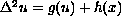.
We show that there is a positive constant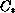, such that if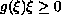for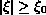and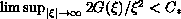, then for all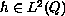with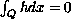, the above equation has a weak solution in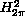.

Published October 31, 2000.
Math Subject Classifications: 35J30, 35B10.
Key Words: periodic solutions, elliptic fourth-order PDE.

Show me the PDF file (136K), TEX file, and other files for this article.

Chen Chang
Division of Mathematics and Statistics
the University of Texas at San Antonio
San Antonio, TX 78249 USA
e-mail: chang@math.utsa.edu